# Create WordCloud with R

A wordcloud is a text mining technique that allows us to visualize most frequently used keywords in a paragraph.

The example wordcloud is shown below :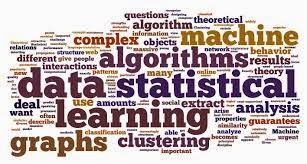Create WordCloud with R Programming
How to create Word Cloud with R

Step 1 : Install the required packages
install.packages("wordcloud")
install.packages("tm")
install.packages("ggplot2")
Note : If these packages are already installed, you don't need to install them again.

Step 2 : Load the above installed packages
library("wordcloud")
library("tm")
library(ggplot2)

Step 3 : Import data into R

Import a single file

Import multiple files from a folder

setwd("C:\\Users\\Deepanshu Bhalla\\Documents\\text mining")
cname <-getwd()
## Number of documents
length(dir(cname))
## list file names
dir(cname)

Note : In the above syntax, "text mining" is a folder name. I have placed all text files in this folder

Step 4 : Locate and load the corpus

If imported a single file

docs<-Corpus(VectorSource(cname[,1]));

If imported multiple files from a folder

docs <- Corpus (DirSource(cname))
docs
summary(docs)
inspect(docs)

Step 5 : Data Cleaning

# Simple Transformation
for (j in seq(docs))
{
docs[[j]] = gsub("(RT|via)((?:\\b\\W*@\\w+)+)", "", docs[[j]])
docs[[j]] = gsub("@\\w+", "", docs[[j]])
docs[[j]] = gsub("http\\w+", "", docs[[j]])
docs[[j]] = gsub("[ \t]{2,}", "", docs[[j]])
docs[[j]] = gsub("^\\s+|\\s+quot;", "", docs[[j]])
docs[[j]] = gsub("[^\x20-\x7E]", "", docs[[j]])
}
# Specify stopwords other than in-bult english stopwords
skipwords = c(stopwords("english"), "system","technology")

kb.tf <- list(weighting = weightTf, stopwords  = skipwords,
removePunctuation = TRUE,
tolower = TRUE,
minWordLength = 4,
removeNumbers = TRUE, stripWhitespace = TRUE,
stemDocument= TRUE)

# term-document matrix
docs <- tm_map(docs, PlainTextDocument)
tdm = TermDocumentMatrix(docs, control = kb.tf)

# convert as matrix
tdm = as.matrix(tdm)

# get word counts in decreasing order
word_freqs = sort(rowSums(tdm), decreasing=TRUE)

# create a data frame with words and their frequencies
dm = data.frame(word=names(word_freqs), freq=word_freqs)

Step 6 : Create WordCloud with R
# Keep wordcloud the same
set.seed(123)

#Plot Histogram
p <- ggplot(subset(dm, freq>10), aes(word, freq))
p <-p+ geom_bar(stat="identity")
p <-p+ theme(axis.text.x=element_text(angle=45, hjust=1))

p

#Plot Wordcloud
wordcloud(dm$word, dm$freq, random.order=FALSE, colors=brewer.pal(6, "Dark2"),min.freq=10, scale=c(4,.2),rot.per=.15,max.words=100)

Note : You can remove sparse terms with the following code :
tdm.frequent = removeSparseTerms(tdm, 0.1)Related PostsDeepanshu founded ListenData with a simple objective - Make analytics easy to understand and follow. He has over 8 years of experience in data science. During his tenure, he has worked with global clients in various domains like Banking, Insurance, Telecom and Human Resource.

23 Responses to "Create WordCloud with R"
1.Awesome article.

1.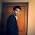Thank you! Glad you liked it :-)

2.I follow your tutorial and I tried to make a word cloud using "I have a dream" text from M. Luther King.

Your post is clear and amazing because I was able to reproduce it. Thank you!

http://www.sthda.com/english/wiki/easyggplot2

3.Hi, I followed all the steps but failed to transform a sentence into a short term. Which is step 5,8
I got the error message:
Error in UseMethod("content", x) :
no applicable method for 'content' applied to an object of class "character"

I can run all Step 1 to Step 4 till now.

Could you help me fix this problem?

1.I have updated the code. That should solve your problem.Thanks!

4.Hi Deepanshu

1.May i know the section of the code which returns this error?

5.Hey, I am using your code, but I can't do my own stopwords. I want to do a wordcloud of a chat and specify some words that shouldn't go into the wordcloud. Can you please tell me how to get my own words into stopwords? Thank you in advance

6.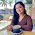Hi !
Great work.
Well, I am trying to create a word-cloud using tweets. But all it shows in the wordcloud is: object,class,status words
Help appreciated.
Thanks

7.Hi Thanks for sharing this.

When I executed step 5 syntax I got below error

Error: unexpected string constant in:
" docs[[j]] = gsub("^\\s+|\\s+quot;, "", docs[[j]])
docs[[j]] = gsub(""
> }
Error: unexpected '}' in "}"

1.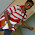The above error was resolved in step 5, but when I executed next set of syntax getting error. Please see below for more details. Can you please let me know why I am getting this error

> tdm = TermDocumentMatrix(docs, control = kb.tf)
Error: inherits(doc, "TextDocument") is not TRUE
>
> # convert as matrix
> tdm = as.matrix(tdm)
>
> # get word counts in decreasing order
> word_freqs = sort(rowSums(tdm), decreasing=TRUE)
>
> # create a data frame with words and their frequencies
> dm = data.frame(word=names(word_freqs), freq=word_freqs)
Error in data.frame(word = names(word_freqs), freq = word_freqs) :

8.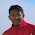This is fantastic!!!

There is a small error in step 5 (instead of " used ;)

for (j in seq(docs))
{
docs[[j]] = gsub("(RT|via)((?:\\b\\W*@\\w+)+)", "", docs[[j]])
docs[[j]] = gsub("@\\w+", "", docs[[j]])
docs[[j]] = gsub("http\\w+", "", docs[[j]])
docs[[j]] = gsub("[ \t]{2,}", "", docs[[j]])

docs[[j]] = gsub("^\\s+|\\s+quot;", "", docs[[j]])

docs[[j]] = gsub("[^\x20-\x7E]", "", docs[[j]])
}

1.Yes George, but in step 5 for next set of syntax I am getting below error, not sure why I am getting this error

> tdm = TermDocumentMatrix(docs, control = kb.tf)
Error: inherits(doc, "TextDocument") is not TRUE
>
> # convert as matrix
> tdm = as.matrix(tdm)
>
> # get word counts in decreasing order
> word_freqs = sort(rowSums(tdm), decreasing=TRUE)
>
> # create a data frame with words and their frequencies
> dm = data.frame(word=names(word_freqs), freq=word_freqs)
Error in data.frame(word = names(word_freqs), freq = word_freqs) :

2.Hi George,

I am sorry i didn't get your point. What exactly you have changed in the code -

for (j in seq(docs))
{
docs[[j]] = gsub("(RT|via)((?:\\b\\W*@\\w+)+)", "", docs[[j]])
docs[[j]] = gsub("@\\w+", "", docs[[j]])
docs[[j]] = gsub("http\\w+", "", docs[[j]])
docs[[j]] = gsub("[ \t]{2,}", "", docs[[j]])
docs[[j]] = gsub("^\\s+|\\s+quot;, "", docs[[j]])
docs[[j]] = gsub("[^\x20-\x7E]", "", docs[[j]])
}

3.Hi Deepanshu,

This code in Step 5
docs[[j]] = gsub("^\\s+|\\s+quot;, "", docs[[j]])

missed the " after "^\\s+|\\s+quot;

9.Issue with the version of tm you are using.

run the following command before running
tdm = TermDocumentMatrix(docs, control = kb.tf)

docs <- tm_map(docs, PlainTextDocument)

1.Thanks a lot George, it does work perfectly.

Just one question about note mentioned, in the syntax and step

Note : You can remove sparse terms with the following code :
tdm.frequent = removeSparseTerms(tdm, 0.1)

What is the use of this?

Thanks a lot for your help

10.One more question, I increased my data points, means I included more comments in .csv file, but got only three word in word cloud.
Whether earlier there were too many words displayed in chart

Why when I added more comments word cloud is showing less words in chart

11.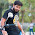i'm using word cloud in shiny , if i select the one data set it have to show the word cloud for corresponding to which i was selected.

1.can any one help me on this issues.

2.can any one help me on this issues.

12.i'm using word cloud in shiny , if i select the one data set it have to show the word cloud for corresponding to which i was selected.

13.Would appreciate it if you could provide a link to the data file(s)?

Next → ← Prev
Love this Post? Spread the Word!
Share